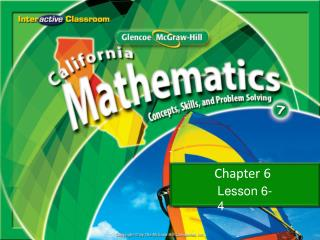Download PresentationSplash ScreenSplash Screen - PowerPoint PPT Presentation

Download PresentationSplash Screen
An Image/Link below is provided (as is) to download presentation

Download Policy: Content on the Website is provided to you AS IS for your information and personal use and may not be sold / licensed / shared on other websites without getting consent from its author. While downloading, if for some reason you are not able to download a presentation, the publisher may have deleted the file from their server.

- - - - - - - - - - - - - - - - - - - - - - - - - - - E N D - - - - - - - - - - - - - - - - - - - - - - - - - - -
Presentation Transcript

1. Splash Screen Chapter 6 Lesson 6-4

2. (over Lesson 6-1) • A • B • C • D Find the value of xin the figure. A.x = 120 B.x = 90 C.x = 60 D.x = 30

3. A. B. C. D. (over Lesson 6-1) • A • B • C • D What is another name for line q?

4. (over Lesson 6-3) • A • B • C • D Find the sum of the interior angle measures of a hexagon. A. 720° B. 540° C. 900° D. 1080°

5. (over Lesson 6-3) • A • B • C • D Find the sum of the interior angle measures of a nonagon. A. 900° B. 1080° C. 1260° D. 1620°

6. Identify congruent polygons. • congruent polygon

7. Standard 7MG3.4Demonstratean understanding of conditions that indicate two geometrical figures are congruent and what congruence means about the relationships between the sides and angles of the two figures.

8. Identify Congruent Polygons Determine whether the trapezoids shown are congruent. If so, name the corresponding parts and write a congruence statement.

9. Identify Congruent Polygons Answer: Since all pairs of corresponding angles and sides are congruent, the two trapezoids are congruent. One congruence statement is trapezoid EFGH≅trapezoid QRST.

10. According to the congruence statement,>Hand <Sare corresponding angles. So, Find Missing Measures Answer: Since m<H= 35°, m<S= 35°.

11. In the figure, Answer: Since FG = 6 centimeters, QR = 6 centimeters.

12. A.B. C.D. If the triangles shown are congruent, which of the following statements is false. • A • B • C • D

13. In the figure, • A • B • C • D A. 50° B. 55° C. 70° D. 75°

14. In the figures, • A • B • C • D A. 3 in. B. 4 in. C. 5 in. D. 6 in.